# DNA Recombination

This imajinary unit is the product of reciprocal complex numbers of the trivial and complex roots that goes recursively with their residual objects. By the nature such as DNA this residual is taken as genetic exchange to generate a better recombination.

Recombination is a process that results in genetic exchange between chromosomes or regions. Recombination counteracts physical linkage between adjacent genes, thereby reducing genetic hitchhiking (Wikipedia).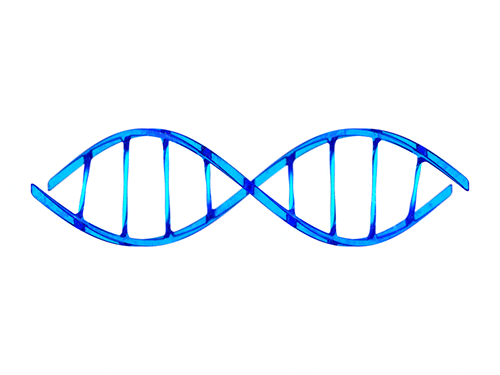Recombination can also generate particular types of mutations if chromosomes are misaligned. Recombination involves the breakage and rejoining of two chromosomes (M and F) to produce two re-arranged chromosomes (C1 and C2).

The result of genes results in more efficient selection, meaning that regions with higher recombination will harbor fewer detrimental mutations, more selectively favored variants, and fewer errors in replication and repair (Wikipedia).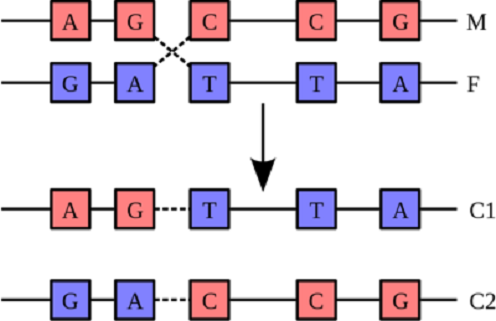Consider that by the True Prime Pairs the 36 is the smallest square expressible as the sum of consecutive prime in two (2) ways of 4 (four) primes and 2 (two) primes.

2nd identity (objects) = 500 x 2/(2+3) = 500 x 2/5 = 200 objects

``````\$True Prime Pairs:
(5,7), (11,13), (17,19)

layer|  i  |   f
-----+-----+---------
|  1  | 5
1  +-----+
|  2  | 7
-----+-----+---  } 36 » 6® by 4 primes
|  3  | 11
2  +-----+
|  4  | 13
-----+-----+---------
|  5  | 17
3  +-----+     } 36 » 6® by 2 primes --------------------
|  6  | 19                                            |
-----+-----+---------                                      |
|
|
layer | node | sub |  i  |  f                               |
------+------+-----+----------
|      |     |  1  | -----------------------   71 by 2 identities √
|      |  1  +-----+                        |
|  1   |     |  2  | (5)                    |
|      |-----+-----+                        |
|      |     |  3  |                        |
1   +------+  2  +-----+----                    |
|      |     |  4  |                        |
|      +-----+-----+                        |
|  2   |     |  5  | (7)                    |
|      |  3  +-----+                        |
|      |     |  6  |                        | 11x
------+------+-----+-----+------      } (36)      |
|      |     |  7  |                        |
|      |  4  +-----+                        |
|  3   |     |  8  | (11)                   |
|      +-----+-----+                        |
|      |     |  9  |                        |
2   +------|  5  +-----+-----                   |
|      |     |  10 |                        |
|      |-----+-----+                        |
|  4   |     |  11 | (13) ------------------   71 by 4 identities √
|      |  6  +-----+
|      |     |  12 |---------------------  (72 - 2) by 1 identity √
------+------+-----+-----+
|      |     |  13 |
|      |  7  +-----+
|  5   |     |  14 | (17)
|      |-----+-----+
|      |     |  15 |
3   +------+  8  +-----+-----
|      |     |  16 |
|      |-----+-----+
|  6   |     |  17 | (19)
|      |  9  +-----+
|      |     |  18 |---------------------  (72 - 4) by 1 identity √
------|------|-----+-----+
``````

Since the position of 19 acts as the exchange base then a new recombination would be re-arranged which is resulting a linkage between 2 (two) and four (4) of prime identities aligned by the residual tensor of 71.

(2 x 32 x 109) − (2 x 32 x 89) = 360

``````layer | node | sub |  i  |  f
------+------+-----+----------                                               ---
|      |     |  1  | 71 (2,3) √ ------------                            |
|      |  1  +-----+                        |                           |
|  1   |     |  2  |                        |                          (5)
|      |-----+-----+                        |                           |
|      |     |  3  |                        |                           |
1   +------+  2  +-----+----                    |                          ---
|      |     |  4  |                        |                           |
|      +-----+-----+                        |                           |
|  2   |     |  5  |                        |                          (7)
|      |  3  +-----+                        |                           |
|      |     |  6  |                        | {6®} √                    |
------+------+-----+-----+------      } (36)      |                          ---
|      |     |  7  |                        |                           |
|      |  4  +-----+                        |                           |
|  3   |     |  8  |                        |                         (11)
|      +-----+-----+                        |                           |
|      |     |  9  |                        |                           |
2   +------|  5  +-----+-----                   |                          ---
|      |     |  10 |                        |                           |
|      |-----+-----+                        |                           |
|  4   |     |  11 | 71 (29,30,31,32) √ ----                          (13)
|      |  6  +-----+                                                    |
|      |     |  12 | {70} (36)                                          |
------+------+-----+-----+------------------                                 ---
|      |     |  13 |                                                    |
|      |  7  +-----+                                                    |
|  5   |     |  14 |                                                  (17)
|      |-----+-----+                                                    |
|      |     |  15 |                                                    |
3   +------+  8  +-----+-----       } (36)                                 ---
|      |     |  16 |                                                    |
|      |-----+-----+                                                    |
|  6   |     |  17 | 100 (10 x 500/100)                                (19)
|      |  9  +-----+                                                    |
|      |     |  18 | 10 x 500/100 (68)                                  |
------|------|-----+-----+------                                             ---
``````

By the 2x25=500/10=50 and 10x10=11+89=100 which is identified from the factorization of 285+286=571 object. Meanwhile 32 is expanded to 32+36=68. So finally the tensor of 71 will be derived to 7×10=68+2=72-2=70 at the center of the scheme.

(10th)th prime = 29th prime = 109 = 110 - 1

``````layer | node | sub |  i  |  f
------+------+-----+----------                                               ---
|      |     |  1  | 71 (2,3) --------------                            |
|      |  1  +-----+                        |                           |
|  1   |     |  2  |                        |                          (5)
|      |-----+-----+                        |                           |
|      |     |  3  |                        |                           |
1   +------+  2  +-----+----                    |                          ---
|      |     |  4  |                        |                           |
|      +-----+-----+                        |                           |
|  2   |     |  5  |                        |                          (7)
|      |  3  +-----+                        |                           |
|      |     |  6  |                        | {6®} = 11 x 30            |
------+------+-----+-----+------      } (36)      |                          ---
|      |     |  7  |                        |                           |
|      |  4  +-----+                        |                           |
|  3   |     |  8  |--- 109 √ ---           | 110-1 by 1 identity √    (11)
|      +-----+-----+             |          |                           |
|      |     |  9  |---- 69 √    | {2®} √   |  71-2 by 1 identity √     |
2   +------|  5  +-----+-----        |          |                          ---
|      |     |  10 |--- 109 √ ---           | 110-1 by 1 identity √     |
|      |-----+-----+                        |                           |
|  4   |     |  11 | 71 (29,30,31,32) ------                          (13)
|      |  6  +-----+                                                    |
|      |     |  12 |                                                    |
------+------+-----+-----+------------------ 329 = 330 - 1 = 309 + 20        ---
|      |     |  13 |                                                    |
|      |  7  +-----+                                                    |
|  5   |     |  14 |                                                  (17)
|      |-----+-----+                                                    |
|      |     |  15 |                                                    |
3   +------+  8  +-----+-----                                              ---
|      |     |  16 |                                                    |
|      |-----+-----+                                                    |
|  6   |     |  17 | 100(50)                                          (19)
168 √ |      |  9  +-----+                                                    |
|     |      |     |  18 | 50(68)                                             |
------|------|-----+-----+------                                             ---
``````

## Palindromic behaviour

The main scheme is two (2) by five (5) objects expressed by three (3) operation of addition (2+5=7), multiplication (2x5=10) and exponentiation (5²=25 and 2^5=32). Here we correlate 11's additive sums with 3960, 7920 and the first 1000 prime numbers.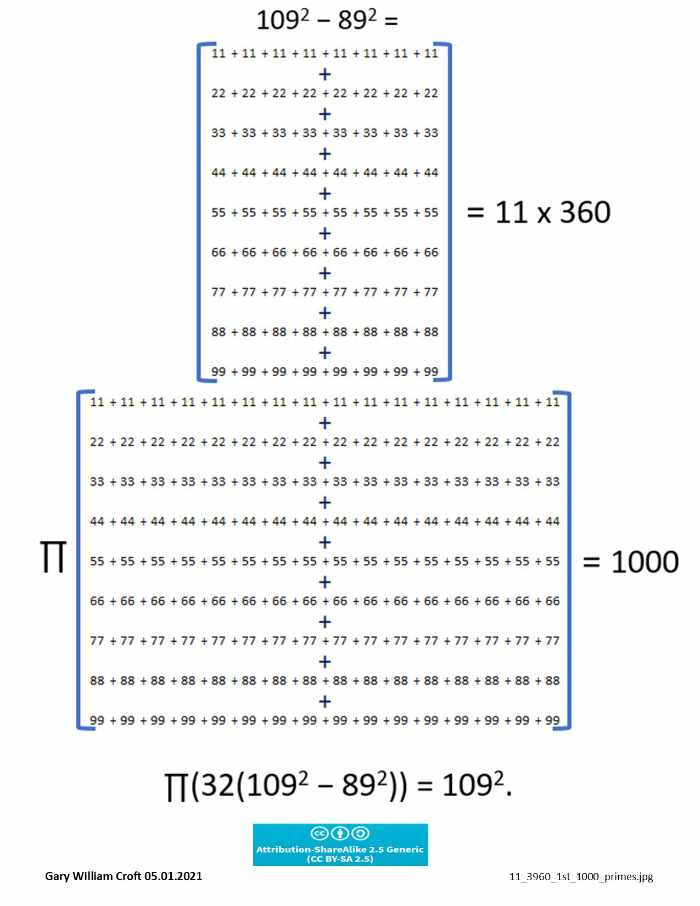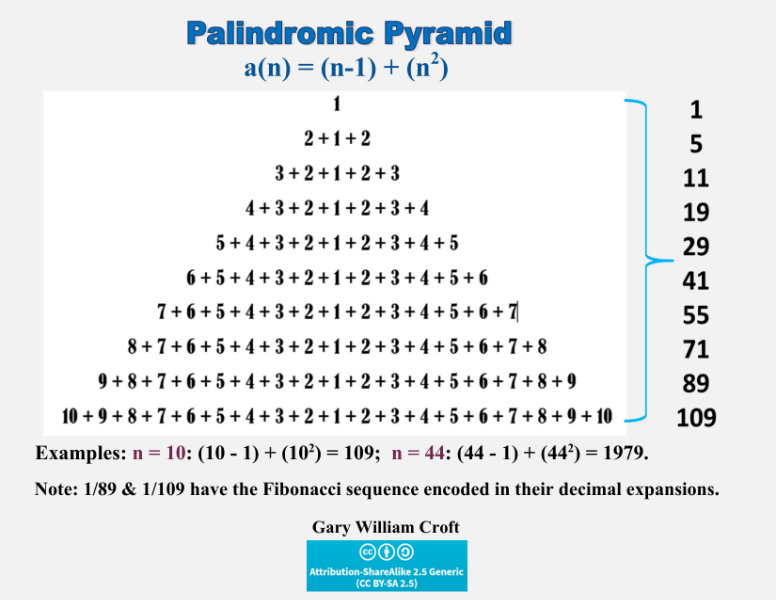In the matrix pictured below, we list the first 24 elements of our domain, take their squares, calculate the modulo 90 congruence and digital roots of each square, and display the digital root factorization dyad for each square (and map their collective bilateral 9 sum symmetry) (Primesdemystified).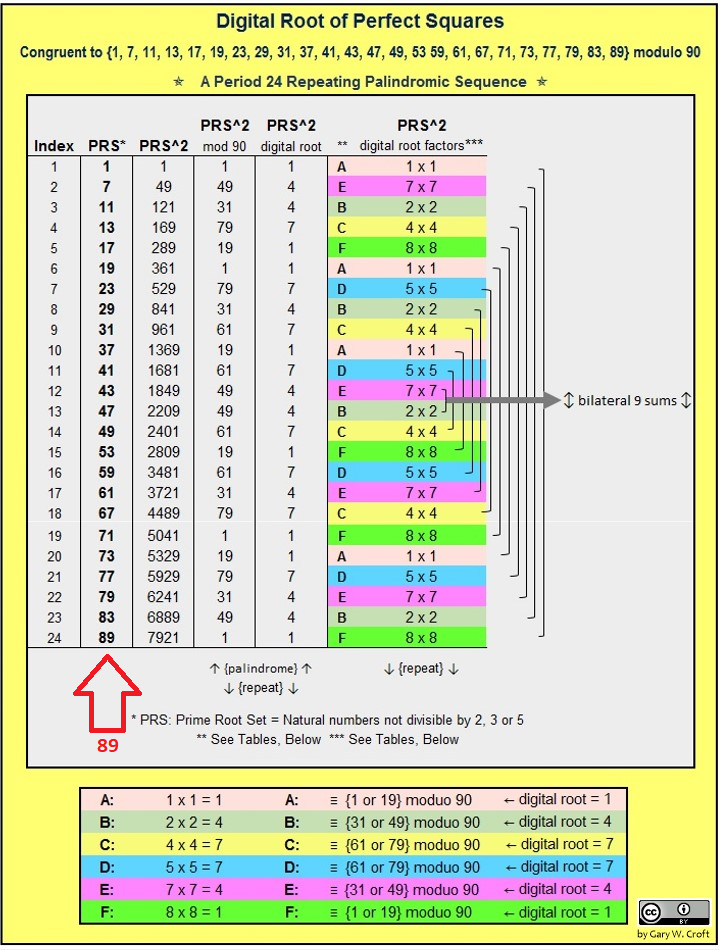## Deep Symmetry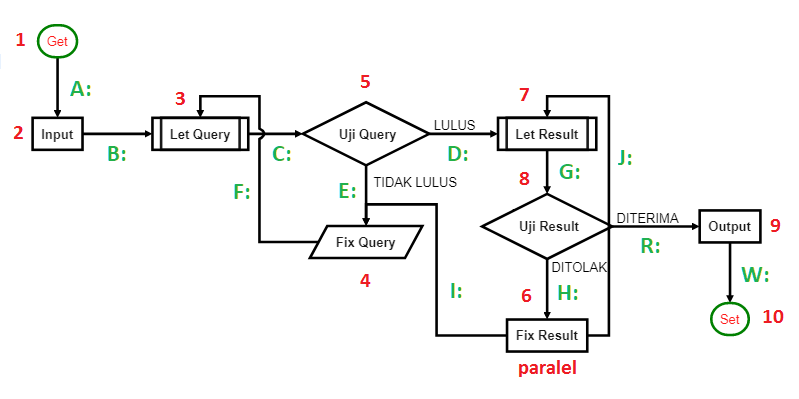Diving deeper yet, here we show the absolute value delta differences of simple reversal sets in relation to primes 89 and 109 contextualized with the first 1000 prime numbers.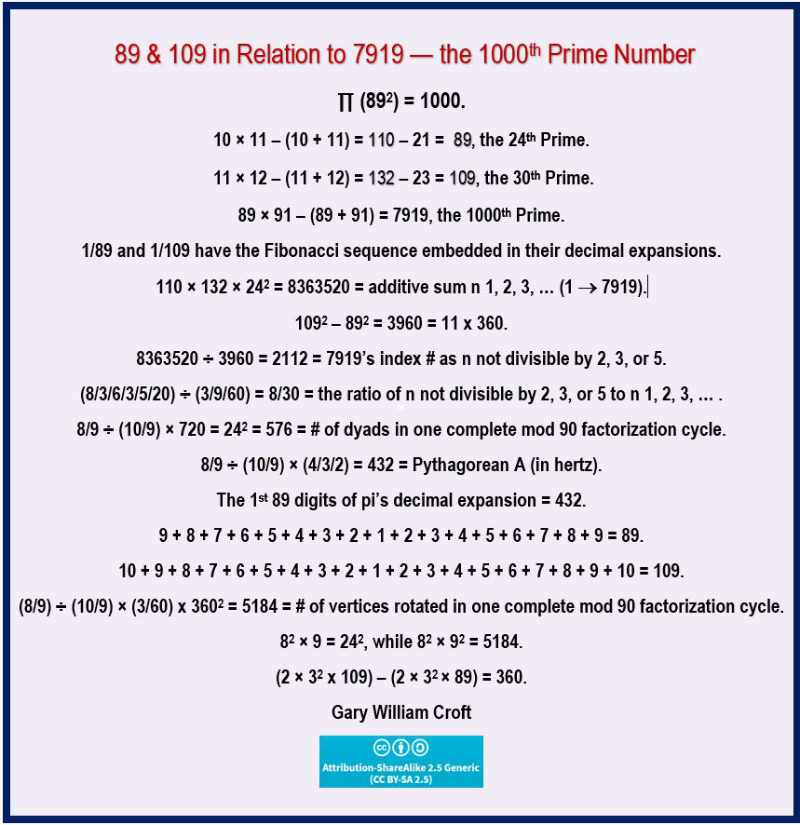Achim Frenzel's popular scientific book, which is illustrated with numerous graphics, is treated as an approach that should definitely be further researched by science. In addition, as a future-oriented project, the book is intended to lead to an interdisciplinary doctorate and therefore refers to its own website as an Internet discussion platform.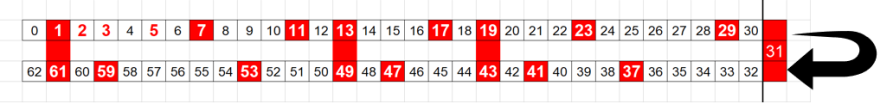An Independent claim is also included for the localization and determination of (I), or their material structures, by graphical representation of base sequences on various media, based on the new assignments and the derived vibrations and amplitudes (Open Patent Data DE19991049059).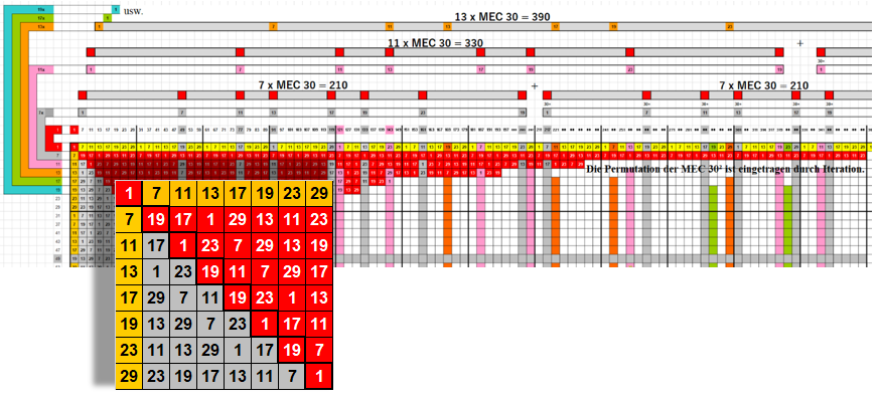See on the left side contains of odd numbers less and equal to 19 while the right is the even number less and equal to 18. By the left side only 9 and 15 that are not primes

Since this bilateral sums of 9 are laid on the prime spiral sieve then the symmetrical behaviour will occur by three (3) rotations of 360. By the 20 objects of 1st prime identity, this 360 will then be separated to 3x100=300 and 3x20=60.

89^2 - 1 = 7921 - 1 = 7920 = 22 x 360 = 66 x 120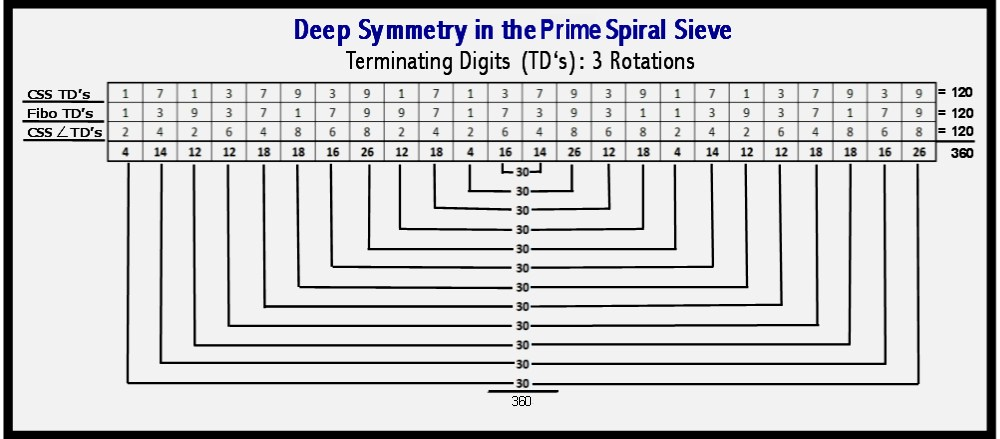By the 500 objects of the 2nd prime identity, the 300 goes to the 29 while the rest of 200 goes as 3rd prime identity to the 89. So the involved objects on the both sides are 300+29=329 and 200+89=289 thus the total will be 329+289=618 objects.